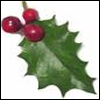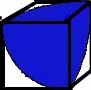#### You may also like### Giant Holly Leaf

Find the perimeter and area of a holly leaf that will not lie flat (it has negative curvature with 'circles' having circumference greater than 2πr).### Quadarc

Given a square ABCD of sides 10 cm, and using the corners as centres, construct four quadrants with radius 10 cm each inside the square. The four arcs intersect at P, Q, R and S. Find the area enclosed by PQRS.### Get Cross

A white cross is placed symmetrically in a red disc with the central square of side length sqrt 2 and the arms of the cross of length 1 unit. What is the area of the disc still showing?

# F'arc'tion

##### Age 14 to 16 ShortChallenge Level

Here is the solution from Christiane Eaves, Alicia Maltby, Kathy Lam, Rachael Evans andFiona Conroy (Y10) The Mount School,York:The total surface area of the cube is $6r^2.$ The area shaded on one face is $\frac{\pi r^2}{4}$ so the total shaded area is $\frac{3\pi r^2}{4}.$

The fraction of the total surface area shaded is thus $\frac{3\pi r^2}{4}$ divided by $6r^2$ which is: $$\frac{3\pi r^2}{4} \times\frac{1}{6r^2}= \frac{\pi}{8}$$
You can find more short problems, arranged by curriculum topic, in our short problems collection.